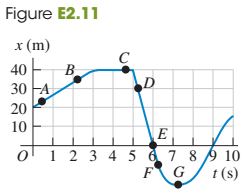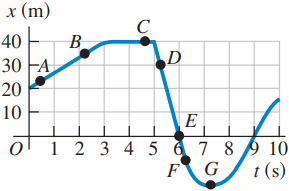×
Get Full Access to University Physics - 13 Edition - Chapter 2 - Problem 11e
Get Full Access to University Physics - 13 Edition - Chapter 2 - Problem 11e

×

# A test car travels in a straight line along the x- axis.ISBN: 9780321675460 31

## Solution for problem 11E Chapter 2

University Physics | 13th Edition

• Textbook Solutions
• 2901 Step-by-step solutions solved by professors and subject experts
• Get 24/7 help from StudySoup virtual teaching assistantsUniversity Physics | 13th Edition

4 5 1 313 Reviews
13
3
Problem 11E

A test car travels in a straight line along the x- axis. The graph in Fig. E2.11 shows the car’s position x as a function of time. Find its instantaneous velocity at points A through G.Step-by-Step Solution:

Step 1 of 4

We can calculate the instantaneous velocity at any point as the slope of the tangent line to the graph at that point.Portion of the curve on which A and B lies appears to be approximately a line segment.

So, we can find the slope of the line, which gives the instantaneous velocity at the point.

Step 2 of 4

Step 3 of 4

##### ISBN: 9780321675460

The answer to “?A test car travels in a straight line along the x- axis. The graph in Fig. E2.11 shows the car’s position x as a function of time. Find its instantaneous velocity at points A through G.” is broken down into a number of easy to follow steps, and 36 words. University Physics was written by and is associated to the ISBN: 9780321675460. The full step-by-step solution to problem: 11E from chapter: 2 was answered by , our top Physics solution expert on 05/06/17, 06:07PM. This full solution covers the following key subjects: Car, its, along, fig, Find. This expansive textbook survival guide covers 26 chapters, and 2929 solutions. This textbook survival guide was created for the textbook: University Physics, edition: 13. Since the solution to 11E from 2 chapter was answered, more than 5111 students have viewed the full step-by-step answer.

## Discover and learn what students are asking

Statistics: Informed Decisions Using Data : Discrete Random Variables
?What are the two requirements for a discrete probability distribution?

Statistics: Informed Decisions Using Data : The Normal Approximation to the Binomial Probability Distribution
?In Problems 5–14, a discrete random variable is given. Assume the probability of the random variable will be approximated using the normal distributio

Statistics: Informed Decisions Using Data : Inference about the Difference between Two Medians: Dependent Samples
?Reaction-Time Experiment Researchers at the University of Mississippi wanted to learn the reaction times of students to different stimuli. In the foll

Unlock Textbook Solution